## Deep Neural Network for Structured Data - Heart Attack Prediction From Scratch

By: Ram on Sep 11, 2020

Preventive and predictive methods can help in managing the devastating effect of heart diseases. In this blog, we aim to show simple steps involved in building a predictive model using the Deep Neural Network method to predict a heart attack.

A detailed overview of Heart Attack Prediction has been discussed here

In this tutorial, we are going to use the heart attack prediction dataset available on Kaggle.

In this heart attack prediction dataset, structured information - factual (e.g. age, height, gender, weight, etc), medical examination results (e.g. BP, Glucose, etc), and behavioral/subjective given by patient (e.g. smoking, taking alcohol, level of physical activity, etc) - is available.

```import pandas as pd cardio = pd.read_csv("cardio_train.csv", sep=";")```

```# Summary Statistics cardio.describe().transpose()```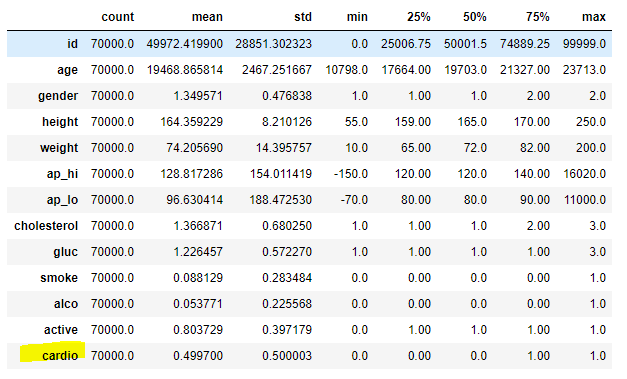### Feature Engineering - Stage 1

A detailed exploratory data analysis has been done to understand data, find the distribution of each of the independent variables/ features, and perform bivariate analysis - relationship between label variable and each of the independent variables/features.

Here are some of the data treatments done and also the features to be created. After that, we create an additional list of features.

```import numpy as np # Age in Years cardio['age_years'] = round(cardio['age']/365,0) # Outlier Treatment: Height cardio['height'] = np.where(cardio['height']>207,207,cardio['height'])```

```# Pressure - High: Category def ap_hi (values):     if values<=120:         return 1     elif 120<values<=200:         return 2     else:         return 3 cardio['ap_hi_cat']=cardio.ap_hi.apply(lambda x: ap_hi(x) ) # Outlier Treatment: ap_hi cardio['ap_hi'] = np.where(cardio['ap_hi']>200,201,cardio['ap_hi'])```

```# Pressure - Low: Category def ap_lo (values):     if values<=50:         return 1     elif 50<values<=120:         return 2     else:         return 3     cardio['ap_lo_cat']=cardio.ap_lo.apply(lambda x: ap_lo(x) )```

```# Capping def capping(series, lowMax, highMin):     if series <lowMax:         return lowMax     elif series>highMin:         return highMin     else:         return series      cardio['ap_hi'] = cardio.ap_hi.apply(lambda x: capping(x,50,120) ) cardio['ap_lo'] = cardio.ap_lo.apply(lambda x: capping(x,50,120) )  # Scale up```

```# Pressure - Low: modulus cardio['ap_lo_mod_10'] = np.where(cardio['ap_lo']%10==0,1,0)```

```# BMI Cal cardio['bmi'] = np.round(cardio['weight']/((cardio['height']/100)*(cardio['height']/100)),0)```

```# Outlier Treatment: BMI import numpy as np cardio['bmi'] = np.where(cardio['bmi']>50,50,cardio['bmi'])```

```# Create multiple group using lamda function def bmicat(values):     if values <=18.5:         return 1     elif 18.5<values<=24.9:         return 2     elif 24.9<values<=29.9:         return 3     else:         return 4      # BMI - Categories cardio['bmi_cat'] = cardio.bmi.apply(lambda x: bmicat(x) ) ```

```# Pressures - difference and ratio cardio['s_d_ratio'] = np.round(np.abs(np.min(cardio['ap_hi']))+1+cardio['ap_hi'],2)/(np.abs(np.min(cardio['ap_lo']))+1+cardio['ap_lo']) cardio['s_d_diff'] = np.round(cardio['ap_hi']-cardio['ap_lo'],2)```

```# Capping Ratio and Difference cardio['s_d_ratio'] = cardio.s_d_ratio.apply(lambda x: capping(x,1.2,1.5) ) cardio['s_d_diff'] = cardio.s_d_diff.apply(lambda x: capping(x,0,50) ) ```

```# EDA - Bivariate Analysis: Dummy Variables cardio['age_above_55'] = np.where(cardio['age_years']>55,1,0) cardio['s_d_diff_above_45'] = np.where(cardio['s_d_diff']>45,1,0) cardio['ap_lo_above_85'] = np.where(cardio['ap_lo']>85,1,0) cardio['ap_hi_above_125'] = np.where(cardio['ap_hi']>125,1,0)```

### Get Variable Type

The type of feature engineering is linked to the variable type. The below function helps in getting type of each feature based on scale of measurement. Based on the feature type, we will create additional features using these features.

```# Find Continuous and Categorical Features def featureType(df):     import numpy as np      from pandas.api.types import is_numeric_dtype```

```    columns = df.columns     rows= len(df)     colTypeBase=[]     colType=[]     for col in columns:         try:             try:                 uniq=len(np.unique(df[col]))             except:                  uniq=len(df.groupby(col)[col].count())             if rows>10:                 if is_numeric_dtype(df[col]):                                          if uniq==1:                         colType.append('Unary')                         colTypeBase.append('Unary')                     elif uniq==2:                         colType.append('Binary')                         colTypeBase.append('Binary')                     elif rows/uniq>3 and uniq>5:                         colType.append('Continuous')                         colTypeBase.append('Continuous')                     else:                         colType.append('Continuous-Ordinal')                         colTypeBase.append('Ordinal')                 else:                     if uniq==1:                         colType.append('Unary')                         colTypeBase.append('Category-Unary')                     elif uniq==2:                         colType.append('Binary')                         colTypeBase.append('Category-Binary')                     else:                         colType.append('Categorical-Nominal')                         colTypeBase.append('Nominal')             else:                 if is_numeric_dtype(df[col]):                     colType.append('Numeric')                     colTypeBase.append('Numeric')                 else:                     colType.append('Non-numeric')                     colTypeBase.append('Non-numeric')         except:             colType.append('Issue')                      # Create dataframe         df_out =pd.DataFrame({'Feature':columns,                           'BaseFeatureType':colTypeBase,                         'AnalysisFeatureType':colType})     # Sort by BaseFeatureType     df_out = df_out.sort_values('BaseFeatureType')     return df_out```

`varTypes=featureType(cardio)  `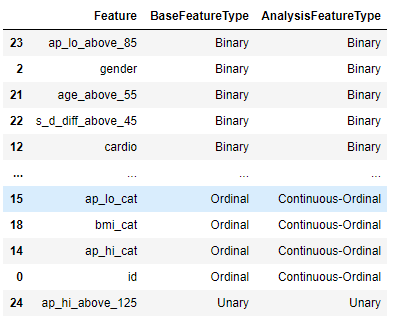### Feature Engineering 2 - Ordinal Variable

Now, we need to create an additional list of variables/features. From an ordinal variable, we can perform one-hot-encoding to create features based on the level of that feature.

The main objective is that we want to use binary variables for building the neural network models. This is mandatory. The only requirement is that all variables should be on the same scale.

We are doing to use the get_dummies function to create one-hot encoding. It automatically deletes the input features.

`# Create dummy variable for each of the ordinal variable level  `

`cardio=pd.get_dummies(data=cardio, columns=['cholesterol','ap_hi_cat','ap_lo_cat','bmi_cat','gluc'] )`

`cardio.head().transpose()`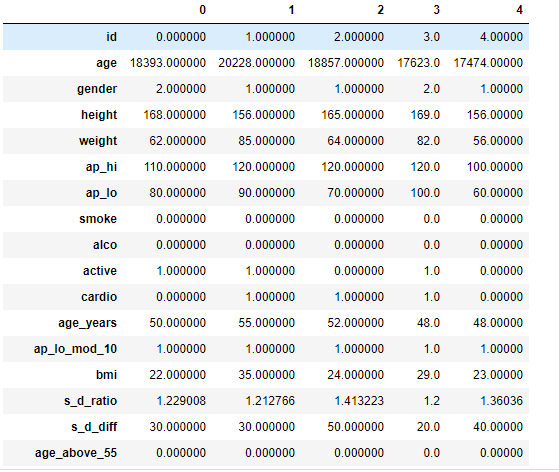### Feature Engineering 2 - Continuous Variable

Now, for continuous features, we need to discretize or create bins before performing one-hot encoding. Using cut(), first created bins - 20 levels (5% each) and then created dummy features - one-hot encoding.

`def ranks20(df, var_list): `

`    for var in var_list:`

`        new_var = var+"_c"`

`        #print(new_var)`

`        # Create 10 grroups`

`        df[new_var] = pd.cut(df[var], bins=20, labels=range(20), right=False)        `

`ranks20(cardio,['ap_lo','ap_hi','weight','age_years','height','bmi','s_d_ratio','s_d_diff'])`

`cardio=pd.get_dummies(data=cardio, columns=['ap_lo_c','ap_hi_c','weight_c','age_years_c','height_c','bmi_c','s_d_ratio_c','s_d_diff_c'] )`

# Find the type of variables

Now, a long list of new features has been created. We need to remove all features but binary features. There are a few unary variables got created which are not relevant. We may want to review why even they got created.

`# Keep only Binary variables`

`binary_features=varTypes.iloc[:,0][varTypes.BaseFeatureType=='Binary']`

`feature_list=binary_features.to_list()`

`cardio_1= cardio.loc[:,feature_list]`

`cardio_1.head()`

### Model Sample Creation

In total 141 features and the label are in the data frame. We can split the data to the label and features data frames. Then we can create testing and training samples.

80% observations in training and 20% in the testing sample.

`# Features - exclude Label column`

`features = cardio_1.drop(['cardio'], axis=1)`

`# Label - select only label column`

`label = pd.DataFrame(cardio_1.loc[:,['cardio']])`

`from sklearn.model_selection import train_test_split`

`features_train, features_test, label_train, label_test = train_test_split(features, label, test_size=0.2, random_state=557)`

`# Check dimensions of both sets.`

`print("Train Features Size:", features_train.shape)`

`print("Test Features Size:", features_test.shape)`

`print("Train Labels Size:", label_train.shape)`

`print("Test Labels Size:", label_test.shape)`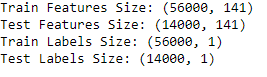### Deep Neural Network for Heart Attack Prediction – Structured Data

Now data samples are ready and a deep neural network model can be defined. We can try a simple neural network with just one input lay and an output layer.

We are also going to add a few dense layers to improve the model performance.

In the first layer, we are defining the number of input features using input_dim. The weight initializer used is normal. There are 141 units in the input layers (each for input feature) and the activation function used is relu.

Dense Layer means each node is connected to all the nodes. In Dropout Layer, based on % input values, it drops those many nodes from the weight updating cycle. It is considered a way to manage overfitting in the neural network.

The output layer has 1 unit with activation function as softmax (generalized for multi-class) or sigmoid considering the label has 2 levels. It is going to calculate the probability between 0 and 1.

Since the output is Binary, binary_crossentropy loss function is used.

```# Define a Neural Network Model from keras.layers import Dense import tensorflow as tf from tensorflow.python.keras.models import Sequential from tensorflow.python.keras.layers import Dense,Dropout from tensorflow.python.keras.optimizers import Adam```

```def NeuralNet(learning_rate):     model = Sequential()     model.add(Dense(141, input_dim=141, kernel_initializer='normal', activation='relu'))     model.add(Dropout(0.2, input_shape=(60,)))     model.add(Dense(10, kernel_initializer='normal', activation='relu'))     model.add(Dense(10, kernel_initializer='normal', activation='relu'))     model.add(Dense(10, kernel_initializer='normal', activation='relu'))     model.add(Dense(1, activation='sigmoid'))     Adam(lr=learning_rate)     model.compile(loss='binary_crossentropy', optimizer='Adam', metrics=['accuracy'])     return model```

```# Build a NN-model, and start training learning_rate = 0.05 model = NeuralNet(learning_rate) print(model.summary())```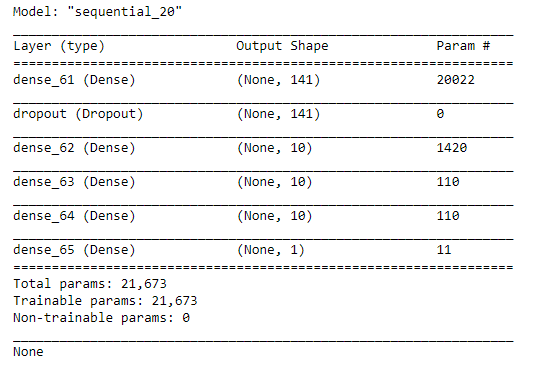### Train the Defined Model

The model can be trained on the training sample created. It will also be tested on the testing sample and compare the accuracy on both the samples.

`# Define the Keras TensorBoard callback.`

`from datetime import datetime`

`from packaging import version`

`import tensorflow as tf`

`from tensorflow import keras`

`import tensorboard`

`import os`

`# Define the Keras TensorBoard callback.`

`log_dir = os.path.join(`

`    "logs",`

`    "fit",`

`    datetime.now().strftime("%Y%m%d-%H%M%S"),`

`)`

`# Storing logs for visualizations`

`tensorboard_callback = keras.callbacks.TensorBoard(log_dir=log_dir)`

`history = model.fit(features_train, label_train, `

`                    validation_data=(features_test, label_test),`

`                    epochs=20,`

`                    batch_size=2000, `

`                    verbose=2,`

`                   callbacks=[tensorboard_callback])`

### Plot Accuracy and Loss

import matplotlib.pyplot as plt
# Plot the model accuracy vs. number of Epochs
plt.plot(history.history['accuracy'])
plt.plot(history.history['val_accuracy'])
plt.title('Model Accuracy')
plt.ylabel('Accuracy')
plt.xlabel('Epochs')
plt.legend(['Train', 'Test'])
plt.show()

# Plot the Loss function vs. number of Epochs
plt.plot(history.history['loss'])
plt.plot(history.history['val_loss'])
plt.title('Model Loss')
plt.ylabel('Loss')
plt.xlabel('Epochs')
plt.legend(['Train', 'Test'])
plt.show()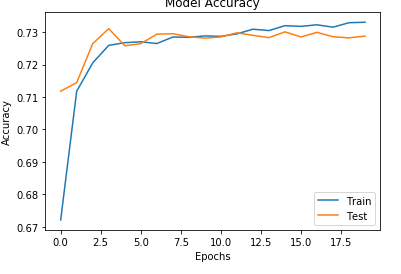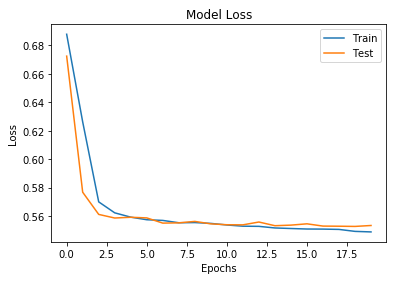Tags
Most PopularJun 18, 2020Jul 23, 2020Jun 19, 2020Jun 19, 2021